@Team 2019-04-18T04:53:47.000000Z 字数 5356 阅读 2104

# 降低一个八度：使用八度卷积减少卷积神经网络的空间冗余

刘源

## 为什么要分离不同频域的信息？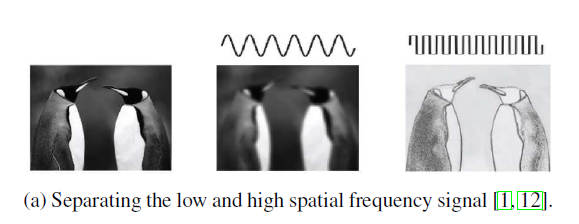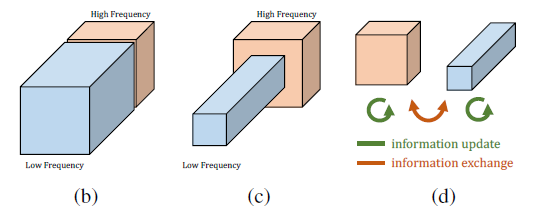## 八度特征表示与八度卷积

### 八度卷积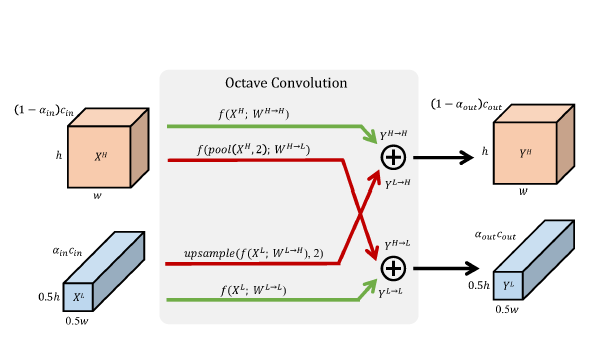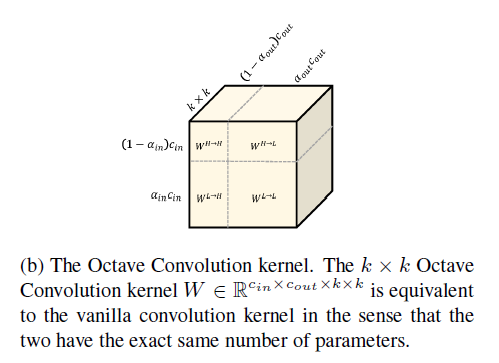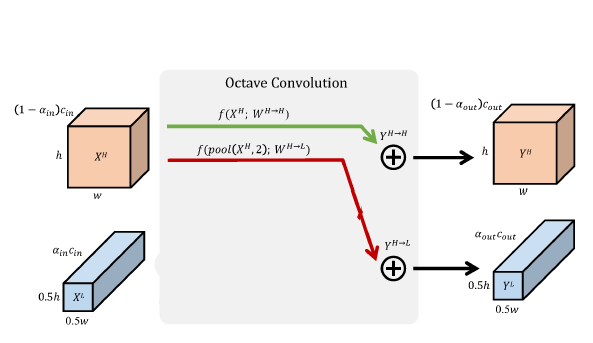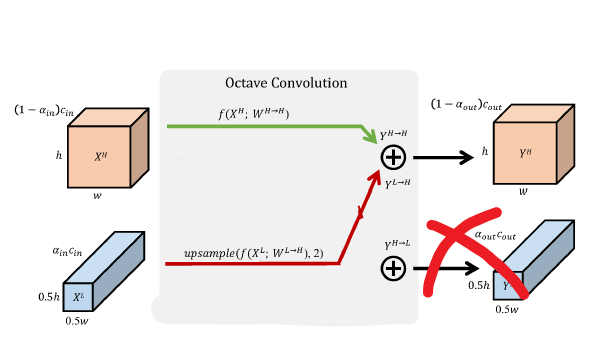### 使用平均池化而不是步长为2的卷积进行下采样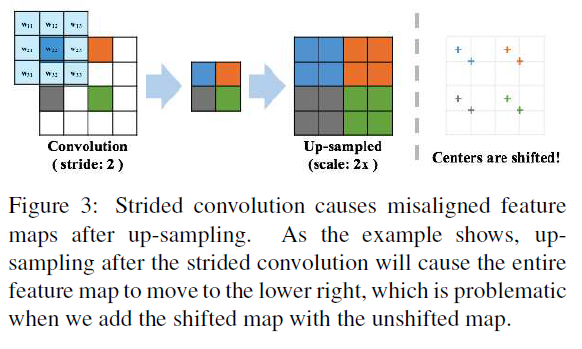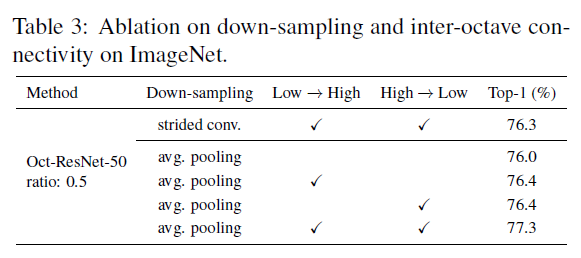## 实验结果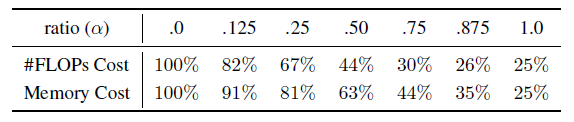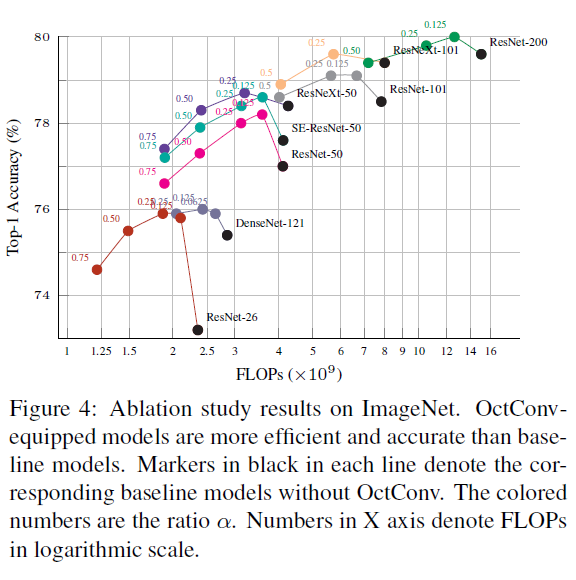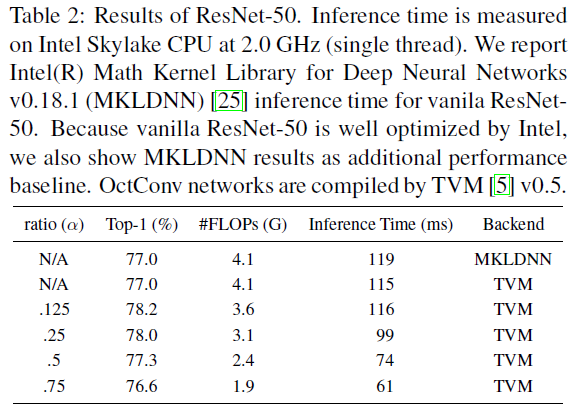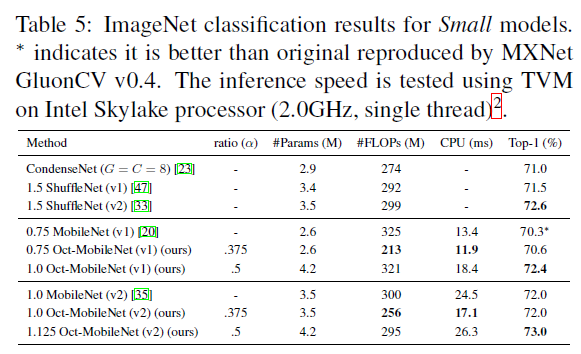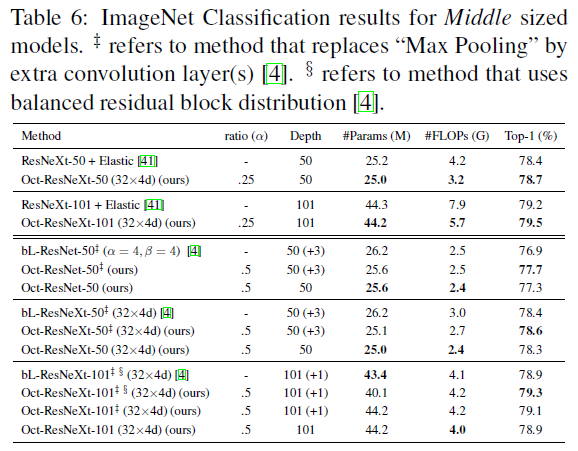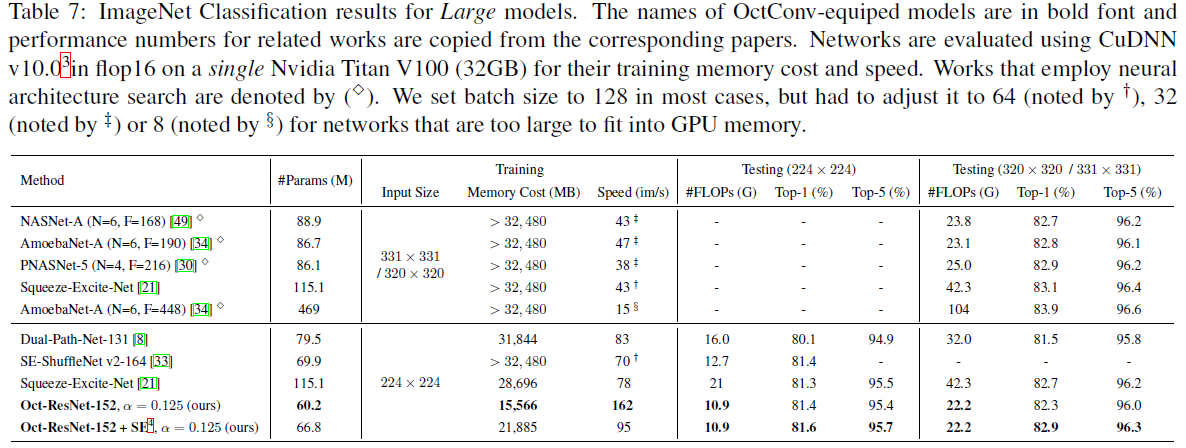## 简单的PyTorch实现（仅供参考）

import math
import torch
import torch.nn as nn
import torch.nn.functional as F
class OctConv2d(nn.Conv2d):
def __init__(
self,
in_channels,
out_channels,
kernel_size,
stride=1,
dilation=1,
groups=1,
bias=True,
alpha_in=0.5,
alpha_out=0.5,):
assert alpha_in >= 0 and alpha_in <= 1
assert alpha_out >= 0 and alpha_out <= 1
super(OctConv2d, self).__init__(in_channels, out_channels,
dilation, groups, bias)
self.avgpool = nn.AvgPool2d(kernel_size=2, stride=2)
self.alpha_in = alpha_in
self.alpha_out = alpha_out
self.inChannelSplitIndex = math.floor(
self.alpha_in * self.in_channels)
self.outChannelSplitIndex = math.floor(
self.alpha_out * self.out_channels)

def forward(self, input):
if not isinstance(input, tuple):
assert self.alpha_in == 0 or self.alpha_in == 1
inputLow = input if self.alpha_in == 1 else None
inputHigh = input if self.alpha_in == 0 else None
else:
inputLow = input
inputHigh = input

output = [0, 0]
# H->H
if self.outChannelSplitIndex != self.out_channels and self.inChannelSplitIndex != self.in_channels:
outputH2H = F.conv2d(
inputHigh,
self.weight[
self.outChannelSplitIndex:,
self.inChannelSplitIndex:,
:,
:],
self.bias[
self.outChannelSplitIndex:],
self.stride,
self.dilation,
self.groups)
output += outputH2H

# H->L
if self.outChannelSplitIndex != 0 and self.inChannelSplitIndex != self.in_channels:
outputH2L = F.conv2d(
self.avgpool(inputHigh),
self.weight[
:self.outChannelSplitIndex,
self.inChannelSplitIndex:,
:,
:],
self.bias[
:self.outChannelSplitIndex],
self.stride,
self.dilation,
self.groups)
output += outputH2L

# L->L
if self.outChannelSplitIndex != 0 and self.inChannelSplitIndex != 0:
outputL2L = F.conv2d(
inputLow,
self.weight[
:self.outChannelSplitIndex,
:self.inChannelSplitIndex,
:,
:],
self.bias[
:self.outChannelSplitIndex],
self.stride,
self.dilation,
self.groups)
output += outputL2L

# L->H
if self.outChannelSplitIndex != self.out_channels and self.inChannelSplitIndex != 0:
outputL2H = F.conv2d(
F.interpolate(inputLow, scale_factor=2),
self.weight[
self.outChannelSplitIndex:,
:self.inChannelSplitIndex,
:,
:],
self.bias[
self.outChannelSplitIndex:],
self.stride,
self.dilation,
self.groups)
output += outputL2H

return tuple(output)• 私有
• 公开
• 删除